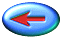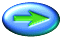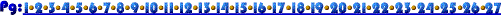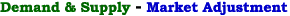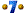Suppose, we have the following demand and supply relationships:
Demand: QD = 120 - 2P
Supply: QS = -30 + P

We found before that, for this market, equilibrium price and quantity are:
P = 50 and
Q = 20

Now, suppose demand shifts back so that the new demand relationship is:
QD2 = 90 - 2P

We can solve for the new equilibrium:
90 - 2P = -30 + P
120 = 3P
P = 40
Q = 10

Our simple demand and supply system confirms the result of our graphical analysis: When demand decreases (shifts back), equilibrium price and quantity both fall.